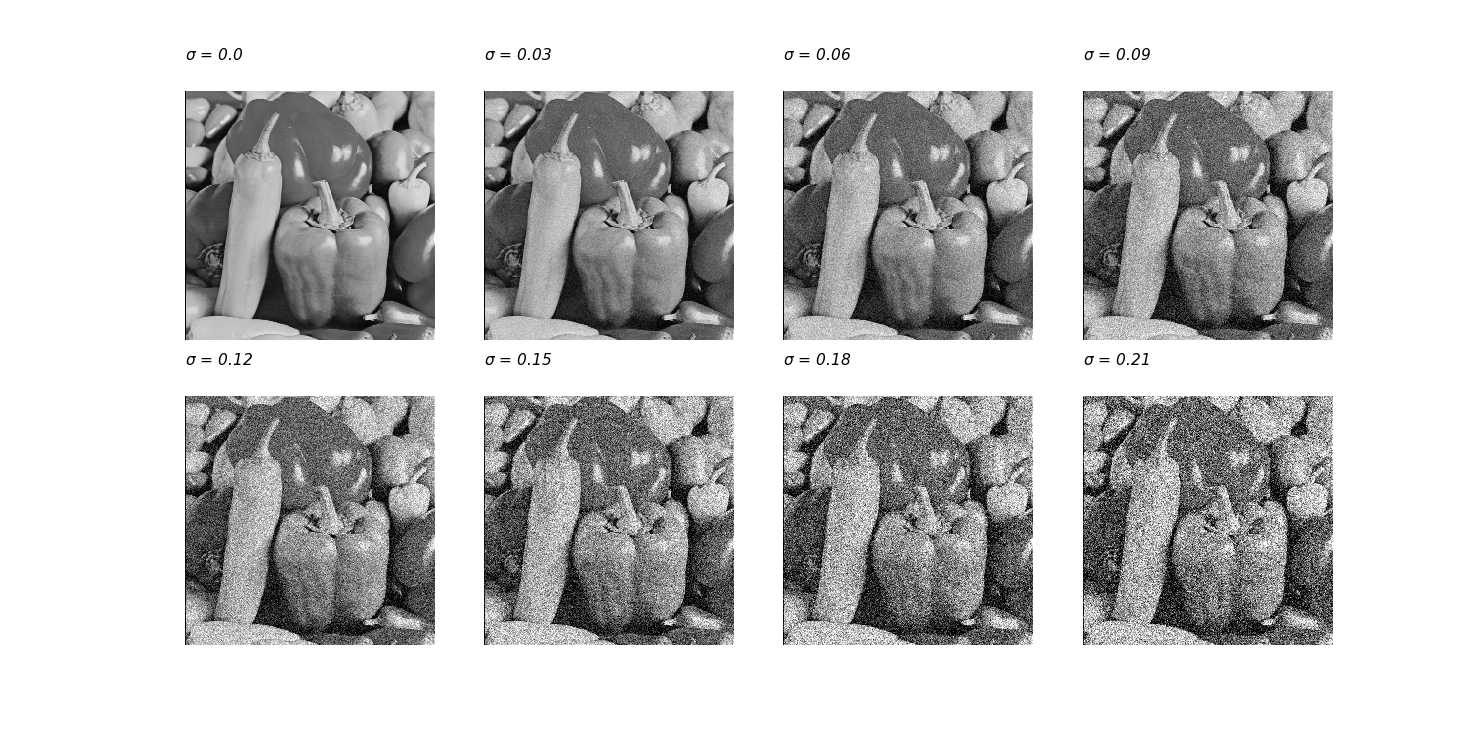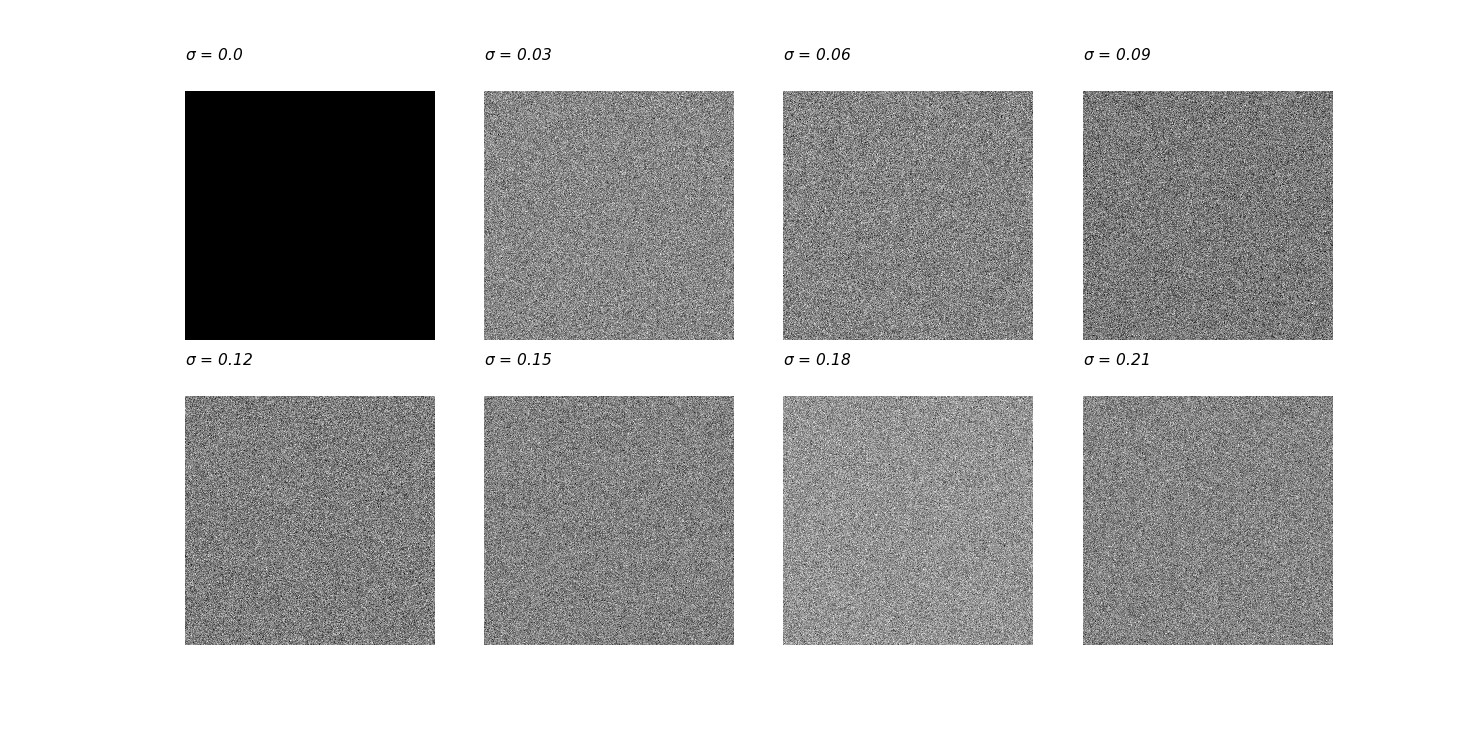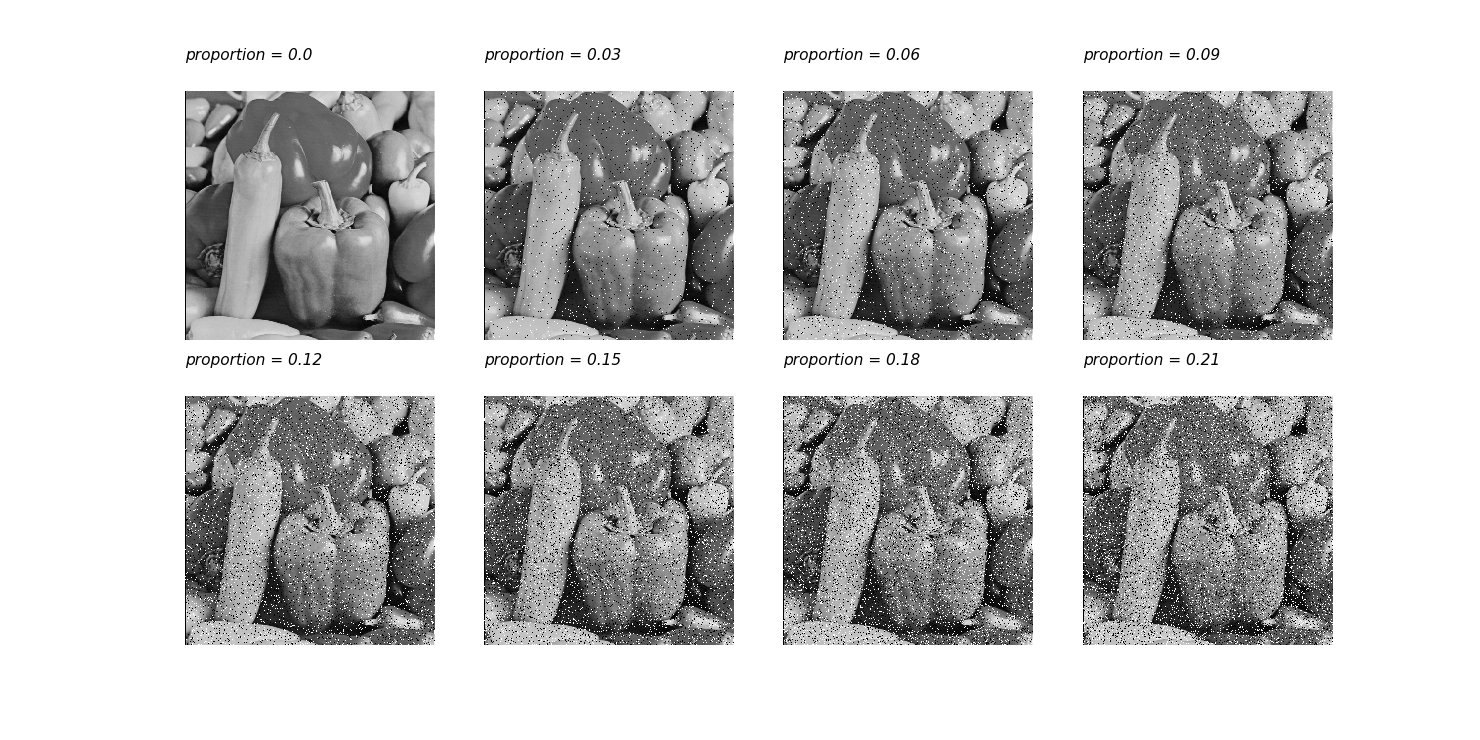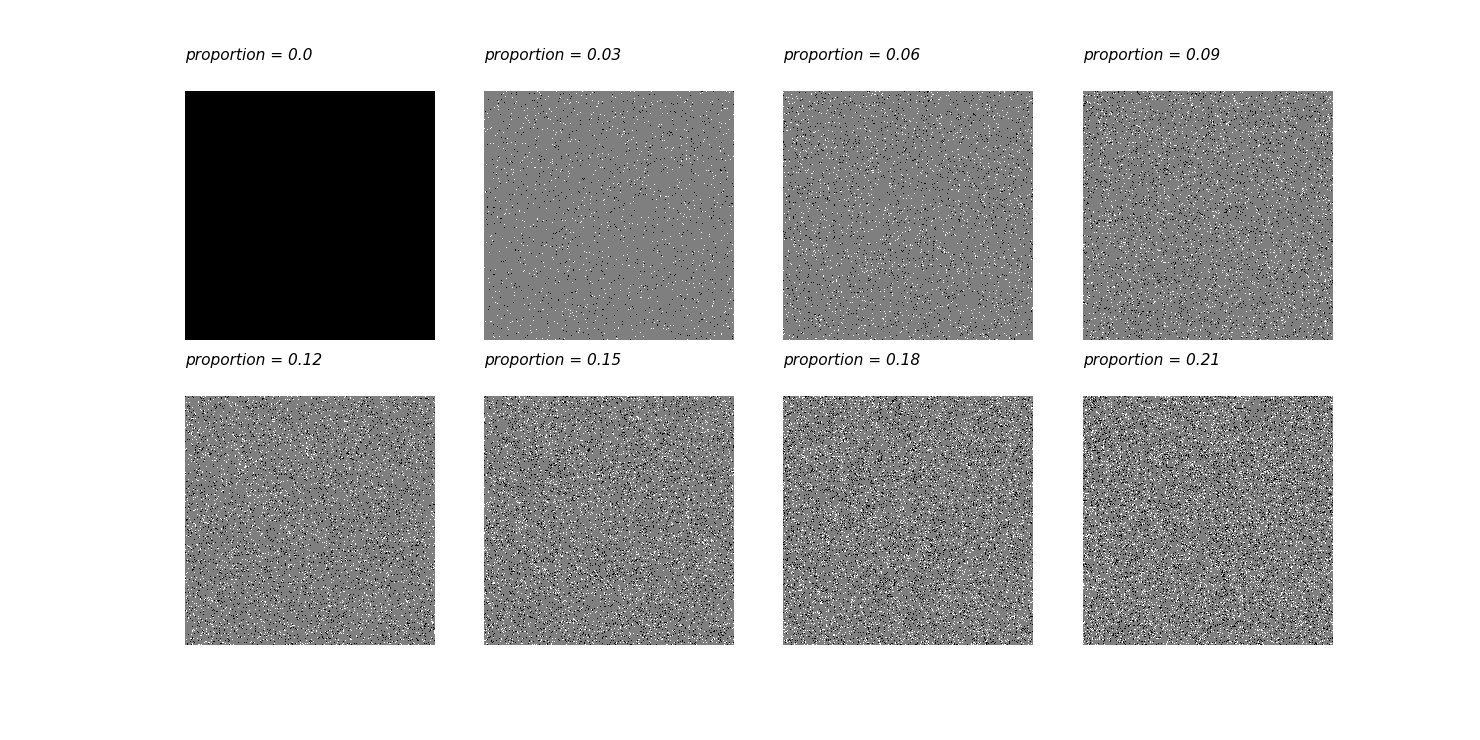高斯噪声、椒盐噪声及其在python中的实现（1）,与,简介,Python,一def saltpepper_noise(image, proportion):
'''
此函数用于给图片添加椒盐噪声
image       : 原始图片
proportion  : 噪声比例
'''
image_copy = image.copy()
# 求得其高宽
img_Y, img_X = image.shape
# 噪声点的 X 坐标
X = np.random.randint(img_X,size=(int(proportion*img_X*img_Y),))
# 噪声点的 Y 坐标
Y = np.random.randint(img_Y,size=(int(proportion*img_X*img_Y),))
# 噪声点的坐标赋值
image_copy[Y, X] = np.random.choice([0, 255], size=(int(proportion*img_X*img_Y),))

# 噪声容器
sp_noise_plate = np.ones_like(image_copy) * 127
# 将噪声给噪声容器
sp_noise_plate[Y, X] = image_copy[Y, X]
return image_copy, sp_noise_plate

def gaussian_noise(img, mean, sigma):
'''
此函数用将产生的高斯噪声加到图片上
传入:
img   :  原图
mean  :  均值
sigma :  标准差
返回:
gaussian_out : 噪声处理后的图片
noise        : 对应的噪声
'''
# 将图片灰度标准化
img = img / 255
# 产生高斯 noise
noise = np.random.normal(mean, sigma, img.shape)
# 将噪声和图片叠加
gaussian_out = img + noise
# 将超过 1 的置 1，低于 0 的置 0
gaussian_out = np.clip(gaussian_out, 0, 1)
# 将图片灰度范围的恢复为 0-255
gaussian_out = np.uint8(gaussian_out*255)
# 将噪声范围搞为 0-255
# noise = np.uint8(noise*255)
return gaussian_out, noisePython Free

QQ：417803890